Rational and Irrational NumbersCustom SearchRATIONAL AND IRRATIONAL NUMBERS Real and imaginary numbers make up the number system of algebra. Imaginary numbers are discussed in chapter 15 of this course. Real numbers are either rational or irrational. The word RATIONAL comes from the word "ratio." A number is rational if it can be expressed as the quotient, or ratio, of two whole numbers. Rational numbers include fractions like 2/7, whole numbers, and radicals if the radical sign is removable. Any whole number is rational. Its denominator is 1. For instance, 8 equals, which is the quotient of two integers. A number likeis rational, since it can be expressed as the quotient of two integers in the form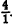. The following are also examples of rational numbers: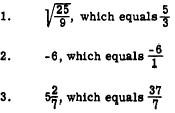Any rational number can be expressed as the quotient of two integers in many ways. For example,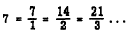An IRRATIONAL number is a real number that cannot be expressed as the ratio of two integers. The numbers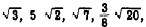and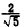are examples of irrational numbers. Rationalizing Denominators Expressions such as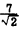andhave irrational numbers in the denominator. If the denominators are changed immediately to decimals, as in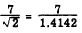the process of evaluating a fraction becomes an exercise in long division. Such a fraction can be evaluated quickly by first changing the denominator to a rational number. Converting a fraction with an irrational number in its denominator to an equivalent fraction with a rational number in the denominator is called RATIONALIZING THE DENOMINATOR. Multiplying a fraction by 1 leaves the value of the fraction unchanged. Since any number divided by itself equals 1, it follows, for example, that If the numerator and denominator of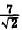are each multiplied by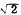, another fraction having the same value is obtained. The result is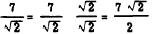The denominator of the new equivalent fraction is 2, which is rational. The decimal value of the fraction is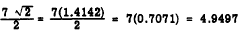To rationalize the denominator in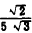we multiply the numerator and denominator by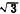. We getPractice problems. Rationalize the denominator in each of the following: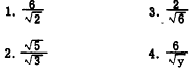Answers:EVALUATING RADICALS Any radical expression has a decimal equivalent which may be exact if the radicand is a rational number. If the radicand is not rational, the root may be expressed as a decimal approximation, but it can never be exact. A procedure similar to long division may be used for calculating square root and cube root, and higher roots may be calculated by means of methods based on logarithms and higher mathematics. Tables of powers and roots have been calculated for use in those scientific fields in which it is frequently necessary to work with roots. SQUARE ROOT PROCESS The arithmetic process for calculation of square root is outlined in the following paragraphs: 1. Begin at the decimal point and mark the number off into groups of two digits each, moving both to the right and to the left from the decimal point. This may leave an odd digit at the right-hand or left-hand end of the number, or both. For example, suppose that the number whose square root we seek is 9025. The number marked off as specified would be as follows:2. Find the greatest number whose square is contained in the left-hand group (90). This number is 9, since the square of 9 is 81. Write 9 above the first group. Square this number (9) place its square below the left-hand group, and subtract, as follows: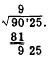Bring down the next group (25) and place it beside the 9, as shown. This is the new dividend (925). 3. Multiply the first digit in the root (9) by 20, obtaining 180 as a trial divisor. This trial divisor is contained in the new dividend (925) five times; thus the second digit of the root appears to be 5. However, this number must be added to the trial divisor to obtain a "true divisor." If the true divisor is then too large to use with the second quotient digit, this digit must be reduced by 1. The procedure for step 3 is illustrated as follows: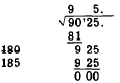The number 180, resulting from the multiplication of 9 by 20,is written as a trial divisor beside the new dividend (925), as shown. The quotient digit (5) is then recorded and the trial divisor is adjusted, becoming 185. The trial quotient (180) is crossed out. 4. The true divisor (185) is multiplied by the second digit (5) and the product is placed below the new dividend (925). This step is shown in the illustration for step 3. When the product in step 4 is subtracted from the new dividend, the difference is 0; thus, in this example, the root is exact.  5. In some problems, the difference is not 0 after all of the digits of the original number have been used to form new dividends. Such problems may be carried further by adding 0’s on the right-hand end of the original number, just as in normal long division. However, in the square root process the 0’s must be added and used in groups of 2. Practice problems. Find the square root of each of the following numbers: 1. 9.61 2. 123.21  3. 0.0025 Answers: 1. 3.1  2. 11.1  3. 0.05Integrated Publishing, Inc. - A (SDVOSB) Service Disabled Veteran Owned Small Business